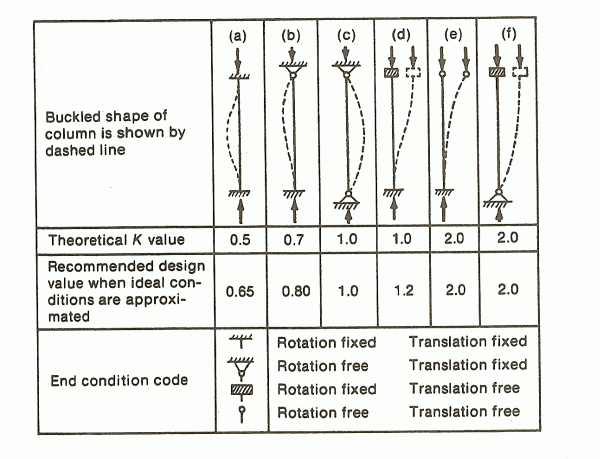HomeAmerican Codes
 3D Render American Canadian General Im-Export
-------------------------------------------------------------------------------------------------------------------------------------------------------------------------------------------------------------------------------------------
Design for Stability
Gu = GL = Slenderness Ratio for non-sway Frames k =

Stiffness reduction factor ( t ) due to column inelasticity:

a).  Pf / Py <= 0.5 (elastic):     t =1.0
b).  Pf / Py > 0.5 (inelastic):    t = 4 (Pf / Py). ( 1 - Pf / Py)

Py = Fy.Ag
Pf - factored axial load on the column, Pf <= 0.85 Py

Methods for Stability Design

Direct Analysis:

1). Use any second-order elastic analysis

3). Use a reduced flexural and axial stiffness to account for influence of inelasticity (EI* = 0.8 t EI, EA* = 0.8 EA)

4). K = 1.0

Effective Length Method: (B2 = D2nd-order / D1st-order <= 1.5)

1). Use any second-order elastic analysis (nominal geometry, stiffness)

Design for Stability